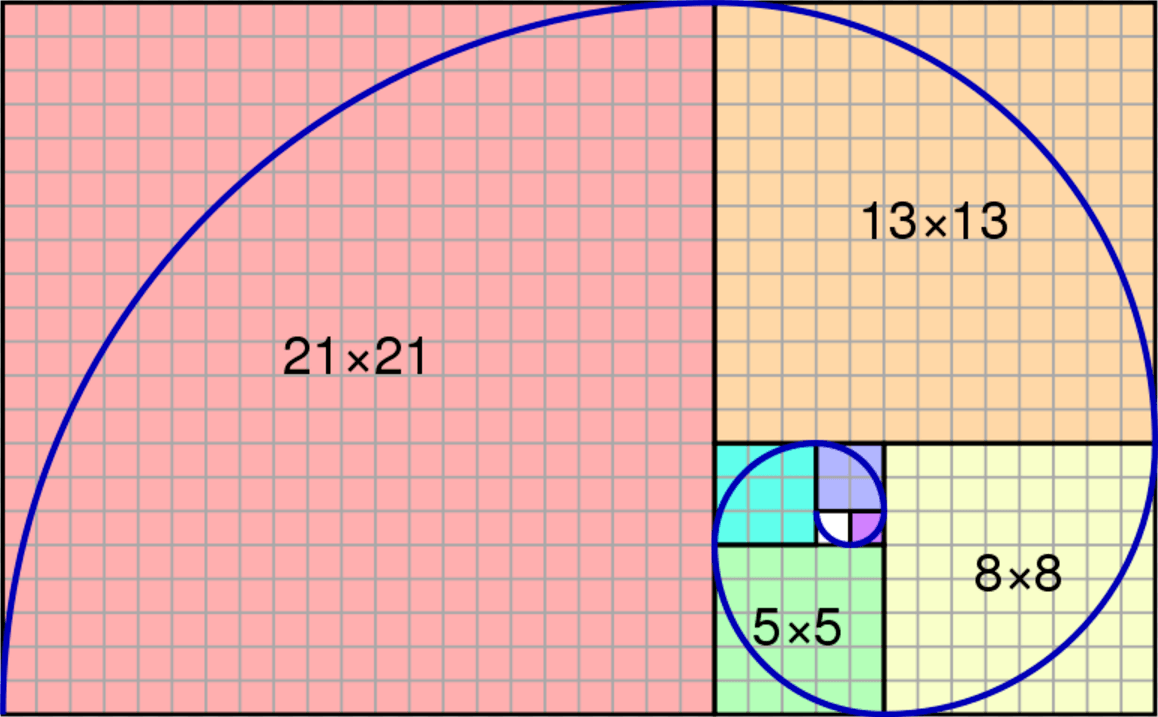# What Is The Fibonacci Sequence? And How It Applies To Agile Development

Beer5020/Shutterstock.com
Summary: The Fibonacci Sequence is a peculiar series of numbers from classical mathematics that has found applications in advanced mathematics, nature, statistics, computer science, and Agile Development. Let’s delve into the origins of the sequence and how it applies to Agile Development.

## What Is The Fibonacci Sequence?

The Fibonacci sequence is a series of numbers where a number is the addition of the last two numbers, starting with 0, and 1.

The Fibonacci Sequence: 0, 1, 1, 2, 3, 5, 8, 13, 21, 34, 55…

Written as a rule, the expression is:

Xn = Xn-1 + Xn-2

The Agile Guide To Agile Development
This guide provides you with a framework for how to transition your team to agile.

### The Fibonacci Spiral And The Golden Ratio

The Fibonacci sequence is often visualized in a graph such as the one in the header of this article. Each of the squares illustrates the area of the next number in the sequence.  The Fibonacci spiral is then drawn inside the squares by connecting the corners of the boxes.The squares fit together perfectly because the ratio between the numbers in the Fibonacci sequence is very close to the golden ratio , which is approximately 1.618034. The larger the numbers in the Fibonacci sequence, the closer the ratio is to the golden ratio.

The spiral and resulting rectangle are also known as the Golden Rectangle .

### The Origins Of The Fibonacci Sequence

Fibbonaci (Leanardo Pisano Bogollo , Fibonacci was his nickname) first introduced the series of numbers known as the Fibonacci sequence in his book Liver Abaci  in 1202. Fibonacci was a member of an important Italian trading family in the 12th and 13th century. Being part of a trading family, mathematics was an integral part of the business. Fibonacci traveled throughout the Middle East and India and was captivated by the mathematical ideas from his travels. His book, Liver Abaci, was a discourse on the mathematical methods in commerce that Fibonacci observed during his travels.

Fibonacci is remembered for two important contributions to Western mathematics:

1. He helped spread the use of Hindu systems of writing numbers in Europe (0,1,2,3,4,5 in place of Roman numerals).
2. The seemingly insignificant series of numbers later named the Fibonacci Sequence after him.

Fibonacci discovered the sequence by posing the following question:

If a pair of rabbits is placed in an enclosed area, how many rabbits will be born there if we assume that every month a pair of rabbits produces another pair and that rabbits begin to bear young two months after their birth?

• Start: At the start no rabbits are born, as the initial pair has not had time to be pregnant and born (0).
• The first month: One pair of rabbits are born (1).
• The second month: Again, one pair of rabbits are born as the new rabbits have not yet matured to bear young (1).
• The third month: Two pairs of rabbits reproduce, and one pair is not ready, so two pairs of rabbits are born (2).
• The fourth month: Three pairs of rabbits reproduce and 2 pairs of rabbits are not ready, so three pairs of rabbits are born (3).
• The fifth month: Five pairs of rabbits produce and three are not ready, so five pairs of rabbits are born (5).
• And so on.

Though Fibonacci’s question concerning the rabbits is an unrealistic scenario, the sequence can be observed in nature, such as in the array of sunflower seeds and other plants, and the shape of galaxies and hurricanes.Sunflower Seeds are a dramatic demonstration the Fibonacci Sequence in nature.

### The Importance of the Fibonacci Sequence

While this series of numbers from this simple brain teaser may seem inconsequential, it has been rediscovered in an astonishing variety of forms, from branches of advanced mathematics  to applications in computer science , statistics , nature , and agile development.

### How Τhe Fibonacci Sequence Ιs Used Ιn Agile Development

Now you may be saying to yourself “That’s nice, but what does it have to do with Agile Development?”, and that’s a great question. What does the Fibonacci Sequence have to do with Agile Development? Interestingly, the Fibonacci’s Sequence is a useful tool for estimating the time to complete tasks.

A big part of managing an Agile team is estimating the time tasks will take to complete. A points system is often used to give a high-level estimate of the scale or size of a specific task. Bigger more complex tasks get more points and smaller tasks get fewer points. Managers can then review and prioritize tasks based upon the assigned scale.

### Using The Fibonacci Sequence With Your Team

To use the Fibonacci Sequence, instruct your team to score tasks from the Fibonacci Sequence up to 21.

1, 2, 3, 5, 8, 13, 21

One being the smallest easiest tasks and twenty-one being large projects. As far as why you should use the Fibonacci sequence instead of, say, t-shirt sizes, check back for my next article on 5 Reasons Using the Fibonacci Sequence Will Make You Better at Estimating Tasks in Agile Development.

And for more on leading an Agile eLearning Development team, check out our sweet eBook guide, The Agile Guide to Agile Development.

#### References

© 2011 - 2023 eLearning Industry